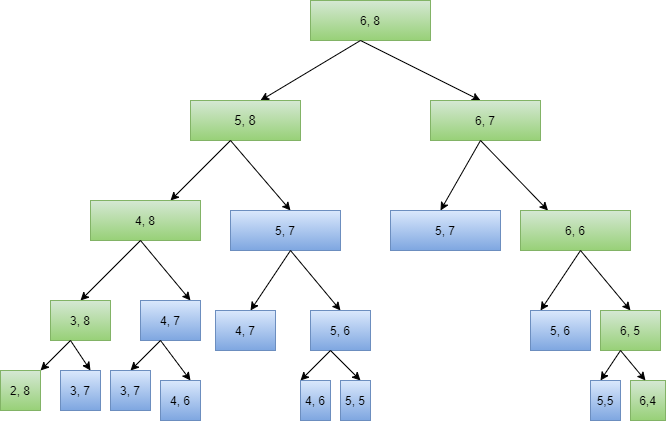# Shortest Common Supersequence

## Introduction.

In this blog, we are going to discuss the approaches for the question Shortest common supersequence. Let’s see the problem statement first: You are given two strings s1 and s2, You have to find the length of the shortest string that can have both strings s1 and s2 as its subsequences.

Let’s understand it better using the example below:

string s1 = “abac”,   string s2 = “cab”

Now your output should be 5 because the string that can accommodate both as its subsequence will be “cabac”.I hope you must be clear with the question. So, let’s move towards solving this problem:-

## Approach#1 Using Recursion

Let’s consider two substrings of length m and n respectively. Now we can have two situations:-

• Both the strings are ending with the same element.

So for finding their shortest common supersequence what we have to do is that we will shorten each string by removing the last element. Then we will be finding the shortest common supersequence of shortened strings and then the removed element will be appended to the shortest common supersequence, that is

SCS(A[1…..m], B[1…...n]) = SCS(A[1….m-1], B[1...n-1]) + A[m]

• Suppose two given strings are not ending at the same character:

In this situation the shortest common supersequence will be the shorter of the two sequences SCS(A[1...m-1], B[1..n]) + A[m] and SCS(A[1...m], B[1...n-1]) + B[n].

Now let’s see this approach in code:

## Complexity Analysis:

Time Complexity: The time complexity will be O(2 ^ (m + n)) where ‘m’ and ‘n’ refer to the length of two strings. Since we are making two recursive calls at each step hence this sums up to exponential time complexity

Space Complexity: The space complexity due to the recursive class will be O(m * n).

This recursive approach for getting the shortest common supersequence exhibits overlapping subproblems.Suppose we have two strings of length 6 and 8 respectively. Let’s see how many overlapping recursive calls are there :-So to eliminate these overlapping recursive calls, we have to solve it using dynamic programming.

## Approach#2 Using Memoization

As you have noticed that we are making many overlapping recursive calls which are costing us in time complexity. So as a solution to this problem we will be taking a 2-D grid for storing our answers. Now we will not be making a recursive call again if this 2-D array is already having the answer to that call. Rest all the steps will be the same as approach 1. Let’s try to give it a shot by yourself then we’ll  be sharing our solution:

## Complexity Analysis:

Time Complexity: The time complexity of this approach will be O(M * N), where ‘M’ and ‘N’ refer to the length of the given strings.

Space Complexity : The space complexity will be O(M * N), where ‘M’ and ‘N’ refer to the length of the given strings.We are consuming this space in storing the results of smaller subproblems.

## Approach#3 Dynamic Programming

In this approach, we will be calculating smaller values of ShortestCommonSupersequence(i, j) first and then we will be building larger values from it.

You will understand all cases clearly by going through below table:

So here I have discussed all the cases. Now try coding it out on your own.

## Complexity Analysis:

Time Complexity: The time complexity of this approach will be O(m * n), where ‘m’ and ‘n’ refer to the length of the given strings.

Space Complexity: The space complexity will be O(m * n), where ‘m’ and ‘n’ refer to the length of the given strings. We are consuming this space in storing the results of smaller subproblems.

Ques 1: What is a subsequence of a string?

Ans 1. A subsequence of a string is obtained by deleting one or more characters from the original string.

Ques 2. Why do we use dynamic programming?

Ans 2. When we solve a problem with recursion, then generally, we make overlapping recursion calls which contribute to poor time complexity. Hence for escaping from these overlapping sub calls, we prefer to write code using DP.

Ques 3. Can I solve this problem in less than O(N * M) time complexity?

Ans 3. No! You can’t because you can see that even in the most optimized approach that is using dynamic programming you need to visit the 2-D matrix at least once.

## Key Takeaways:

In this blog, we discussed two approaches for the problem of the shortest common supersequence. You can see how we started from exponential time complexity and then moved on to optimizing it. Remember this is how you should present your solution in an interview.

If you want to practice some super important coding problems for your interview, then I have an awesome platform CodeStudio for you. Keep practicing and growing. Happy Coding!

By: Deeksha Sharma